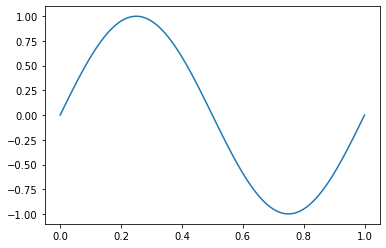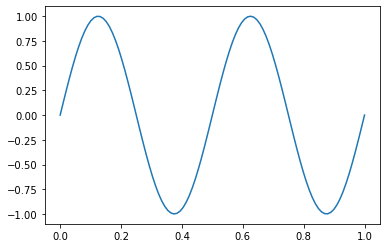# Managing state¶

The notebook is an interactive computing environment. It lets you execute code in a step-by step fashion. This is powerful for thinking through a problem and taking your next steps based on what you see or do in the cell above. But it can lead to some challenging, non-intuitive behaviours if you run cells out of order or multiple times. In this notebook, we capture some “gotchas” and tips for avoiding tying your notebook in a knot. If you have ideas or experiences to contribute here, contributions to this repository are welcome!

```import numpy as np
import matplotlib.pyplot as plt
```

## Variable assignment¶

Sometimes as you are working, you might do simple computations, updates to a variable. One way to do this is to just over-write the variable name. The danger here is that if you re-run the second or third cell below, the value will update again, which may not be intended.

If you have a large notebook and update a parameter above, re-run the cells below, this can be easy to miss and generate non-intuitive results.

```a = 2
```
```a *= 2
print(a)
```
```4
```
```a = a*2
print(a)
```
```8
```

A safer way to do this operation is to create a new variable with a different name. No matter how many times we execute the second cell below, the value will always be `4`

```a = 2
```
```a_times_2 = a*2
print(a_times_2)
```
```4
```

## Scope of functions¶

The scope of variables assigned at the top level in the notebook (e.g. not inside a function or class) is global. This means you can still use them inside of a function. In the second cell below, we create a sine wave with a given frequency.

```frequency = 1
```
```def sketchy_sine_wave(x):
return np.sin(2*np.pi*frequency*x)
```
```x = np.linspace(0, 1, 100)
plt.plot(x, sketchy_sine_wave(x));
```If we change the value of `frequency`, then the the behaviour of `sine_wave` changes. So even though the second cell has code that is identical to the cell above, the output is different. This can be confusing!

```frequency = 2
```
```plt.plot(x, sketchy_sine_wave(x));
```A safer approach is to keep the scope of variables inside a function local to that function and assign default-values to parameters as appropriate. This will also allow you to much more easily pull functions / classes that you write in the notebook into a python file to create a module if you want to later re-use that code.

```def safer_sine_wave(x, frequency=1):
return np.sin(2*np.pi*frequency*x)
```